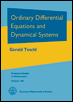Book
Graduate Studies in Mathematics, Volume 140, Amer. Math. Soc., Providence, 2012.

## Ordinary Differential Equations and Dynamical Systems

### Gerald Teschl

Abstract
This book provides an introduction to ordinary differential equations and dynamical systems. We start with some simple examples of explicitly solvable equations. Then we prove the fundamental results concerning the initial value problem: existence, uniqueness, extensibility, dependence on initial conditions. Furthermore we consider linear equations, the Floquet theorem, and the autonomous linear flow.

Then we establish the Frobenius method for linear equations in the complex domain and investigate Sturm-Liouville type boundary value problems including oscillation theory.

Next we introduce the concept of a dynamical system and discuss stability including the stable manifold and the Hartman-Grobman theorem for both continuous and discrete systems.

We prove the Poincare-Bendixson theorem and investigate several examples of planar systems from classical mechanics, ecology, and electrical engineering. Moreover, attractors, Hamiltonian systems, the KAM theorem, and periodic solutions are discussed as well.

Finally, there is an introduction to chaos. Beginning with the basics for iterated interval maps and ending with the Smale-Birkhoff theorem and the Melnikov method for homoclinic orbits.

MSC: 34-01, 37-01
Keywords: Ordinary differential equations, Dynamical systems, Sturm-Liouville equations.

Ordering DetailsPublisher: American Mathematical Society Series: Graduate Studies in Mathematics, ISSN: 1065-7338 Volume: 140 Publication Year: 2012 ISBN: 0-8218-8328-3 / 978-0-8218-8328-0 Paging: 356 pp; hardcover List Price: \$64 Member Price: \$51.20 Itemcode: GSM/140
Order from AMS Book Store

A Solutions Manual is available electronically for instructors only. Please send email to textbooks@ams.org for more information.

A chinese translation has been published by China Machine Press (ISBN 78-7-111-32710-3).

The AMS has granted the permisson to make an online edition available as pdf (4.0M). Permission is granted to retrieve and store a single copy for personal use only. If you like this book and want to support the idea of online versions, please consider buying this book. A solutions manual with full solutions to all problems is available for instructors only from the AMS.

The chinese edition is available as pdf (6.5M) version. In addition, there is a notebook (1M) with the Mathematica code from the text plus some further extensions. To solve complex equations via the power series method you also need my package DiscreteMath`DiffEqs`.

Any comments and bug reports are welcome! There is also an errata containing known errors.

Part 1: Classical theory
1. Introduction
1. Newtons equations
2. Classification of differential equations
3. First order equations
4. Finding explicit solutions
5. Qualitative analysis of first order equations
6. Qualitative analysis of first order periodic equations
2. Initial value problems
1. Fixed point theorems
2. The basic existence and uniqueness result
3. Some extensions
4. Dependence on the initial condition
5. Regular perturbation theory
6. Extensibility of solutions
7. Euler's method and the Peano theorem
3. Linear equations
1. The matrix exponential
2. Linear autonomous first order systems
3. Linear autonomous equations of order n
4. General linear first order systems
5. Linear equations of order n
6. Periodic linear systems
7. Perturbed linear first order systems
8. Appendix: Jordan canonical form
4. Differential equations in the complex domain
1. The basic existence and uniqueness result
2. The Frobenius method for second order equations
3. Linear systems with singularities
4. The Frobenius method
5. Boundary value problems
1. Introduction
2. Symmetric compact operators
3. Sturm-Liouville equations
4. Regular Sturm-Liouville problems
5. Oscillation theory
6. Periodic Sturm-Liouville equations
6. Part 2: Dynamical systems
7. Dynamical systems
1. Dynamical systems
2. The flow of an autonomous equation
3. Orbits and invariant sets
4. The Poincaré map
5. Stability of fixed points
6. Stability via Liapunov's method
7. Newton's equation in one dimension
8. Planar dynamical systems
1. Examples from ecology
2. Examples from electrical engineering
3. The Poincaré-Bendixson theorem
9. Higher dimensional dynamical systems
1. Attracting sets
2. The Lorenz equation
3. Hamiltonian mechanics
4. Completely integrable Hamiltonian systems
5. The Kepler problem
6. The KAM theorem
10. Local behavior near fixed points
1. Stability of linear systems
2. Stable and unstable manifolds
3. The Hartman-Grobman theorem
4. Appendix: Integral equations
11. Part 3: Chaos
12. Discrete dynamical systems
1. The logistic equation
2. Fixed and periodic points
3. Linear difference equations
4. Local behavior near fixed points
13. Discrete dynamical systems in one dimension
1. Period doubling
2. Sarkovskii's theorem
3. On the definition of chaos
4. Cantor sets and the tent map
5. Symbolic dynamics
6. Strange attractors/repellors and fractal sets
7. Homoclinic orbits as source for chaos
14. Periodic solutions
1. Stability of periodic solutions
2. The Poincare map
3. Stable and unstable manifolds
4. Melnikov's method for autonomous perturbations
5. Melnikov's method for nonautonomous perturbations
15. Chaos in higher dimensional systems
1. The Smale horseshoe
2. The Smale-Birkhoff homoclinic theorem
3. Melnikov's method for homoclinic orbits
16. Bibliography
Glossary of notations
Index